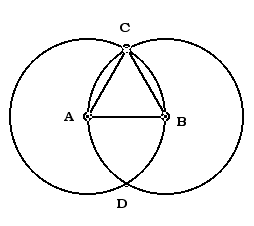Monday, February 25, 2008

3Archimedes explains in his work “On the Measurement of the Circle” that the whole number ratio 153:265 accurately approximates the irrational or incomeasurable ratio of the /3 as the measure of the fish. Archimedes also explains that this approximation was well known to his contemporaries: “It required no word of explanation at all.” This ratio 153:265 defines the measurement of the rhombus in its height and length within the central point of the vesica.
Mathematically, 153 is a triangular number, more precisely it is the sum of the integer numbers from 1 to 17 inclusive; more significantly, 153 also has the rare property that it is the sum of the cubes of its own digits (i.e. 153 = 1x1x1 + 5x5x5 + 3x3x3). In the time of Pythagoras, 153 was most significant for being one of the two numbers in the closest fraction known, at the time, to the true value of the square root of 3, the fraction in question being 265/153 (the difference between this and the square root of 3 is merely 0.000025......). The ratio of 153:265 was consequently known throughout the Hellenic world as the measure of the fish.

Plato states in Timaeus: “But two things cannot be rightly put together without a third; there must be some bond of union between them. And the fairest bond is that which makes the most complete fusion of itself and the things which it combines; and proportion is best adapted to effect such a union. For whenever in any three numbers, whether cube or square, there is a mean, which is to the last term what the first term is to it; and again, when the mean is to the first term as the last term is to the mean -- then the mean becoming first and last, and the first and last both becoming means, they will all of them of necessity come to be the same, and having become the same with one another will be all one.”

In John's Gospel, Jesus miraculously helps his disciples land a large catch of 153 fish: "Simon Peter climbed aboard and dragged the net ashore. It was full of large fish, 153, but even with so many the net was not torn." (John 21:11) - It's an occult meaning. In fact, 153 is a sacred number associated with the vesica piscis or 'vessel of the fish', an ancient Pythagorean symbol. The ratio of length to height of this shape is 265:153, and is known as the 'measure of the fish'. It is a powerful mathematical tool, being the nearest whole number approximation of the square root of three and the controlling ratio of the equilateral triangle.